# Simulating Life, Cells, and randomness

Cellular computations or automation have often been used to answer the question of whether randomness actually exists in nature as a principle or is simply a result of interactions between most basic units of complexity.

Cellular automata can be thought of as the most basic representation of computation in a complex environment. Some of the representations are actually found to be Turing compute. Imagine creating a most basic form of computation life to understand and imitate the complex behavior of natural processes and the multi-level interactions between different entities. The initial ideas were worked on by Von Neumann, however, the science of CAs gained popularity in the 1970s by Conway’s game of life and in the 1980s by Wolfram's work on CAs. Cellular automata can be seen as an alternative to study highly complex and dynamic systems where simpler models cannot completely explain the physical behavior or reality, in essence, it's learning through recreating the smaller model of computations. Examples studying and simulating hurricane wind patterns instead of solving fluid dynamics differential equations.

Each CAs can be thought of as a Turing state machine and the rules can be thought of a set of program which runs on that machine. Obviously, the rules are generally simpler and are created to understand the dynamics of the system. For example, the Turing complete rule 110 is just the binary (01101110) applied on a 1-D input.

The system is generally set up to run in grids, however, in theory, at least some of the forms of CAs are capable of running any program given enough time and resources.

The most basic cellular automata can be simple an on and off-state machine on a 1-D plane. Let's take an example of 10 rows and the rule to be the following

X(t) = X(t-1) + 1 mod 2

`# let n rows 1-D gridn = 10x = np.zeros(n)print(x)for i in range(2, n):# my rule is simple reverse the output of the previous cell  x[i] = (x[i-1] + 1) % 2print(x)# The output is purely determinstic # Output is [0. 0. 0. 0. 0. 0. 0. 0. 0. 0.] #[0. 1. 0. 1. 0. 1. 0. 1. 0. 1.]`

Lets build a 2-D version of the above. We can create a rule such as a summing of all three neighbor values in the row and output in the next row. We will pass each row through a window of 3 cells in each iteration

`rows = 5cols = 11array = np.zeros((rows, cols), dtype=np.uint8)array[0, 5] = 1print(array)def plot_CA(array):plt.imshow(array, cmap='Blues', interpolation='none')plot_CA(array)#Let Array be my 2-D spacedef step(array, i):rows, cols = array.shaperow = array[i-1]for j in range(1, cols):elts = row[j-1:j+2]array[i, j] = sum(elts) % 2# Now lets iterate over 1 stepstep(array, 1)#Complete Iterationfor i in range(1, rows):     step(array, i)`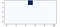At Step 0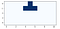Step 1 Iteration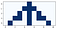Surprisingly a complicated pattern for simple rule

A more efficient way of implementing rules is using cross-correlation this is very similar to convolution in deep nets.

Implementing the program with correlation

`rows = 5cols = 11array = np.zeros((rows, cols), dtype=np.uint8)array[0, 5] = 1def step(array, i, window=[1,1,1]):  row = array[i-1]  c = np.correlate(row, window, mode='same')  array[i] = c % 2for i in range(1, rows):step2(array, i)plot_ca(array)`

Creating a function to convert rules to binary 1-D inputs for passing in the correlation function

`def make_table(rule):"""Make the table for a given CA rule.rule: int 0-255returns: array of 8 0s and 1s"""rule = np.array([rule], dtype=np.uint8)# convert to binary and reverse the arraytable = np.unpackbits(rule)[::-1]return table`

Implementing Wolfram approach.

In his book “A new kind of Science” wolfram created four classes of celluar automata. Surprisingly, many of CAs showed randomness born out of strict deterministic simple rules. The resulting appearance and behaviour is completely the product of interaction of simple units creating a more complex system

Class 1: These produce simple and pre-determined outcomes, based on the rules set. These represent simple functions in mathematics.

Class 2: These produce pre-determined yet complex outcomes. These can be thought of as generally complicated mathematical structures but without any randomness

Class 3: These produces a completely random response from a surprisingly pre-determined set of rules and behave like pseudo-random number generators

Class 4: The most popular class of CAs, these produce both deterministic and with elements of randomness, similar to physical systems. These are Turing complete CAs, capable of computations, similar to Conway’s game of life.

Lets create a basic CA class

`class Cell1D:    """Represents a 1-D a cellular automaton"""    def __init__(self, rule, n, m=None):        """Initializes the CA.        rule: integer        n: number of rows        m: number of columns        Attributes:        table:  rule dictionary that maps from triple to next state.        array:  the numpy array that contains the data.        next:   the index of the next empty row.        """        self.table = make_table(rule)        self.n = n        self.m = 2*n + 1 if m is None else m        self.array = np.zeros((n, self.m), dtype=np.int8)        self.next = 0    def start_single(self):        """Starts with one cell in the middle of the top row."""        self.array[0, self.m//2] = 1        self.next += 1    def start_random(self):        """Start with random values in the top row."""        self.array = np.random.random(self.m).round()        self.next += 1    def start_string(self, s):        """Start with values from a string of 1s and 0s."""        # TODO: Check string length        self.array = np.array([int(x) for x in s])        self.next += 1    def loop(self, steps=1):        """Executes the given number of time steps."""        for i in range(steps):            self.step()    def step(self):        """Executes one time step by computing the next row of the array."""        a = self.array        i = self.next        window = [4, 2, 1]        c = np.correlate(a[i-1], window, mode='same')        a[i] = self.table[c]        self.next += 1    def draw(self, start=0, end=None):        """Draws the CA using pyplot.imshow.        start: index of the first column to be shown        end: index of the last column to be shown        """        a = self.array[:, start:end]        plt.imshow(a, cmap='Blues', alpha=0.7)                # turn off axis tick marks        plt.xticks([])        plt.yticks([])`

Now the drawing function

`def draw_ca(rule, n=32):    """Makes and draw a 1D CA with a given rule.        rule: int rule number    n: number of rows    """    ca = Cell1D(rule, n)    ca.start_single()    ca.loop(n-1)    ca.draw()`

Class 1 representations

`draw_ca(rule=1, n=50)draw_ca(rule=3, n=50)`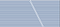Rule 3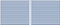Rule 1

Class 2 CAs

`#now for a little more complexitydraw_ca(rule=50, n=50)`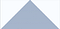The cells arrange themselves and produce a perfectly symmetrical and titled pattern

Class 3, random patterns and space ships, we use rule 30 as an example.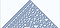A random pattern with Spaceships. Randomness will increase with size of the grid

Class 4, deterministic and yet random. (Rule 110)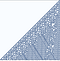The pattern evolves as the size of grid increases grid of 200 x 200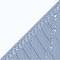Grid of 300 x 300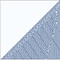More spaceships appear randomly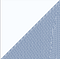Structures of spaceships appear at random yet overall have a repeating pattern

CAs is essentially representing computation from the basic principles, none of the structures are programmed. Rule 110 has been studied extensively and found to be Turing complete and hence can be applied on various types of arrays representing different programs or data sets.

Similar ideas of randomness can be used to extend to any computational systems small or large. Large computational systems such as AI/GPT-3 or biological systems, will show more variability in the final output.

Wolfram noted that some systems cannot be completely explained using a general theory but must be simulated and studied with experimentation to understand the outputs.